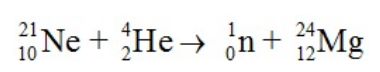Ch.19 - Nuclear ChemistryWorksheetSee all chapters
 Ch.1 - Intro to General Chemistry 2hrs & 53mins 0% complete Worksheet Ch.2 - Atoms & Elements 2hrs & 49mins 0% complete Worksheet Ch.3 - Chemical Reactions 3hrs & 25mins 0% complete Worksheet BONUS: Lab Techniques and Procedures 1hr & 38mins 0% complete Worksheet BONUS: Mathematical Operations and Functions 47mins 0% complete Worksheet Ch.4 - Chemical Quantities & Aqueous Reactions 3hrs & 55mins 0% complete Worksheet Ch.5 - Gases 3hrs & 47mins 0% complete Worksheet Ch.6 - Thermochemistry 2hrs & 28mins 0% complete Worksheet Ch.7 - Quantum Mechanics 2hrs & 35mins 0% complete Worksheet Ch.8 - Periodic Properties of the Elements 1hr & 57mins 0% complete Worksheet Ch.9 - Bonding & Molecular Structure 2hrs & 5mins 0% complete Worksheet Ch.10 - Molecular Shapes & Valence Bond Theory 1hr & 31mins 0% complete Worksheet Ch.11 - Liquids, Solids & Intermolecular Forces 3hrs & 40mins 0% complete Worksheet Ch.12 - Solutions 2hrs & 17mins 0% complete Worksheet Ch.13 - Chemical Kinetics 2hrs & 22mins 0% complete Worksheet Ch.14 - Chemical Equilibrium 2hrs & 26mins 0% complete Worksheet Ch.15 - Acid and Base Equilibrium 4hrs & 42mins 0% complete Worksheet Ch.16 - Aqueous Equilibrium 3hrs & 48mins 0% complete Worksheet Ch. 17 - Chemical Thermodynamics 1hr & 44mins 0% complete Worksheet Ch.18 - Electrochemistry 2hrs & 58mins 0% complete Worksheet Ch.19 - Nuclear Chemistry 1hr & 33mins 0% complete Worksheet Ch.20 - Organic Chemistry 3hrs 0% complete Worksheet Ch.22 - Chemistry of the Nonmetals 2hrs & 1min 0% complete Worksheet Ch.23 - Transition Metals and Coordination Compounds 1hr & 54mins 0% complete Worksheet

## Mass Defect

See all sections
Sections
Band of Stability
Magic Numbers
First Order Half Life

# Solution: How much energy is released in the following nuclear reaction?Mass of the 21Ne = 20.993849 amu; Mass of 4He = 4.002604 amu;Mass of a neutron = 1.008665; Mass of 24Mg = 23.985045(a) 9.51 x 107 kJ.mol(b

###### Problem

How much energy is released in the following nuclear reaction?

Mass of the 21Ne = 20.993849 amu; Mass of 4He = 4.002604 amu;

Mass of a neutron = 1.008665; Mass of 24Mg = 23.985045

(a) 9.51 x 107 kJ.mol

(b) 471 kJ.mol

(c) 9.22 x 1010 kJ/mol

(d) 7.65 x 104 kJ/mol

(e) 2.47 x 108 kJ/mol###### Solution

We’re being asked to calculate the energy released for the following nuclear reaction:

${}_{\mathbf{10}}{}^{\mathbf{21}}\mathbit{N}\mathbit{e}\mathbf{+}{}_{\mathbf{2}}{}^{\mathbf{4}}\mathbit{H}\mathbit{e}\mathbf{\to }{}_{\mathbf{0}}{}^{\mathbf{1}}\mathbit{n}\mathbf{+}{}_{\mathbf{12}}{}^{\mathbf{24}}\mathbit{M}\mathbit{g}$

To calculate the energy released for the reaction, we’re going to use the following steps:

Step 1: Calculate the mass defect (Δm).
Step 2: Calculate the mass defect (Δm) in kg.
Step 3: Calculate the energy released (E).

Step 1: Calculate the mass defect (Δm).

Given:

View the complete written solution...